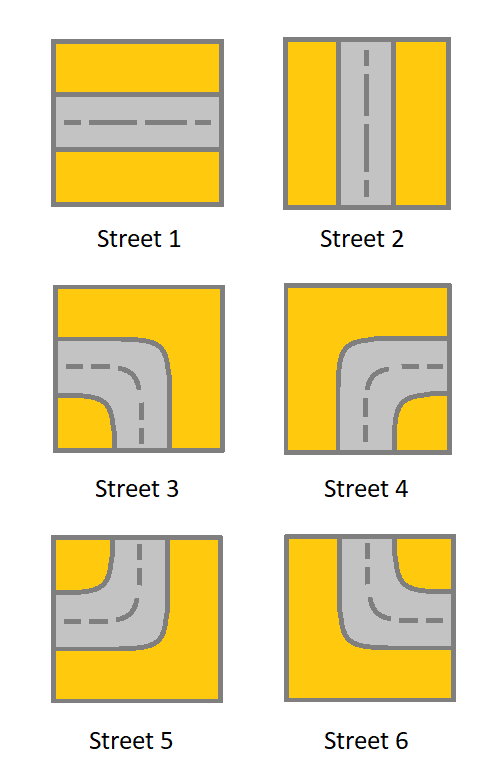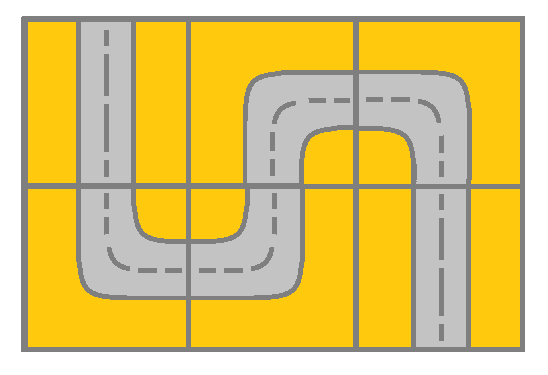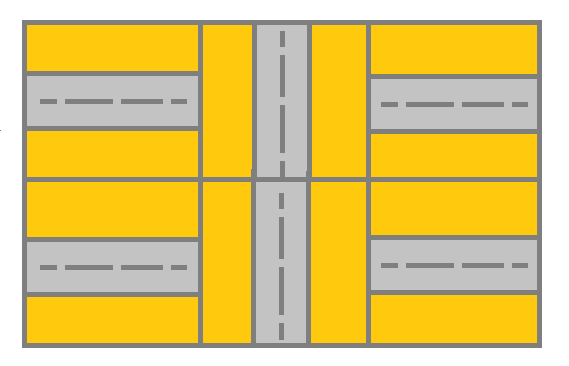# 1391. Check if There is a Valid Path in a Grid

Given a m x n `grid`. Each cell of the `grid` represents a street. The street of `grid[i][j]` can be:
• 1 which means a street connecting the left cell and the right cell.
• 2 which means a street connecting the upper cell and the lower cell.
• 3 which means a street connecting the left cell and the lower cell.
• 4 which means a street connecting the right cell and the lower cell.
• 5 which means a street connecting the left cell and the upper cell.
• 6 which means a street connecting the right cell and the upper cell.You will initially start at the street of the upper-left cell `(0,0)`. A valid path in the grid is a path which starts from the upper left cell `(0,0)` and ends at the bottom-right cell `(m - 1, n - 1)`. The path should only follow the streets.

Notice that you are not allowed to change any street.

Return true if there is a valid path in the grid or false otherwise.

Example 1:```Input: grid = [[2,4,3],[6,5,2]]
Output: true
Explanation: As shown you can start at cell (0, 0) and visit all the cells of the grid to reach (m - 1, n - 1).
```

Example 2:```Input: grid = [[1,2,1],[1,2,1]]
Output: false
Explanation: As shown you the street at cell (0, 0) is not connected with any street of any other cell and you will get stuck at cell (0, 0)
```

Example 3:

```Input: grid = [[1,1,2]]
Output: false
Explanation: You will get stuck at cell (0, 1) and you cannot reach cell (0, 2).
```

Example 4:

```Input: grid = [[1,1,1,1,1,1,3]]
Output: true
```

Example 5:

```Input: grid = [,,,,,,]
Output: true
```

Constraints:

• `m == grid.length`
• `n == grid[i].length`
• `1 <= m, n <= 300`
• `1 <= grid[i][j] <= 6`

Medium

Normal

Robinhood

### Solution(Chinese):

LEETCODE 1391. Check if There is a Valid Path in a Grid 解题思路分析